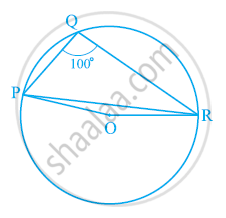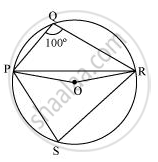# In the given figure, ∠PQR = 100°, where P, Q and R are points on a circle with centre O. Find ∠OPR. - Mathematics

In the given figure, ∠PQR = 100°, where P, Q and R are points on a circle with centre O. Find ∠OPR.#### SolutionConsider PR as a chord of the circle.

Take any point S on the major arc of the circle.

∠PQR + ∠PSR = 180° (Opposite angles of a cyclic quadrilateral)

⇒ ∠PSR = 180° − 100° = 80°

We know that the angle subtended by an arc at the centre is double the angle subtended by it at any point on the remaining part of the circle.

∴ ∠POR = 2∠PSR = 2 (80°) = 160°

In ΔPOR,

OP = OR (Radii of the same circle)

∴ ∠OPR = ∠ORP (Angles opposite to equal sides of a triangle)

∠OPR + ∠ORP + ∠POR = 180° (Angle sum property of a triangle)

2 ∠OPR + 160° = 180°

2 ∠OPR = 180° − 160° = 20º

∠OPR = 10°

Is there an error in this question or solution?
Chapter 10: Circles - Exercise 10.5 [Page 185]

#### APPEARS IN

NCERT Class 9 Maths
Chapter 10 Circles
Exercise 10.5 | Q 3 | Page 185

Share